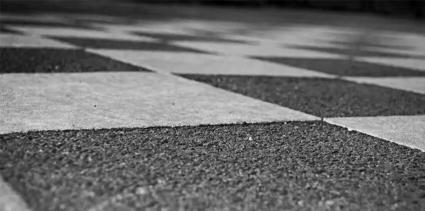# Spatial Autocorrelation and Moran’s I in GISA checkerboard pattern is an example where Moran’s I is -1 because dissimilar values are next to each other.

Spatial autocorrelation in GIS helps understand the degree to which one object is similar to other nearby objects. Moran’s I (Index) measures spatial autocorrelation.

Geographer Waldo R. Tobler’s stated in the first law of geography:

“Everything is related to everything else, but near things are more related than distant things.”

• Positive spatial autocorrelation is when similar values cluster together on a map.
• Negative spatial autocorrelation is when dissimilar values cluster together on a map.

Spatial autocorrelation measures how close objects are in comparison with other close objects. Moran’s I can be classified as positive, negative, and with no spatial auto-correlation.

## Why is Spatial Autocorrelation Important?

One of the main reasons why spatial auto-correlation is important is because statistics rely on observations being independent of one another. If autocorrelation exists in a map, then this violates the fact that observations are independent of one another.

Another potential application is analyzing clusters and dispersion of ecology and disease.

• Is the disease an isolated case?
• Is it clustered or spreading with dispersion?

These trends can be better understood using spatial autocorrelation analysis.

### Positive Spatial Autocorrelation Example

Positive spatial autocorrelation occurs when Moran’s I is close to +1. This means values cluster together. For example, elevation datasets have similar elevation values close to each other.

There is clustering in the land cover image above. This clustered pattern generates a Moran’s I of 0.60. The z-score of 4.95 indicates there is a less than 1% likelihood that this clustered pattern could be the result of a random choice.

### Negative Spatial Autocorrelation Example

Negative spatial auto-correlation occurs when Moran’s I is near -1. A checkerboard is an example where Moran’s I is -1 because dissimilar values are next to each other. A value of 0 for Moran’s I typically indicates no autocorrelation.

Using the spatial autocorrelation tool in ArcGIS, the checkerboard pattern generates a Moran’s index of -1.00 with a z-score of -7.59.

(Remember that the z-score indicates the statistical significance given the number of features in the dataset).

This checkerboard pattern has a less than 1% likelihood that it is the result of a random choice. If you want to test this statistical technique, try GeoDa software for this and more.

### What’s Next: Spatial Dependency

Spatial autocorrelation indicates if there is clustering or dispersion in a map. While a positive Moran’s I hints at data is clustered, a negative Moran’s I implies data is dispersed.

If you’ve tested this spatial autocorrelation guide, try to master spatial statistics widely used statistics in GIS: